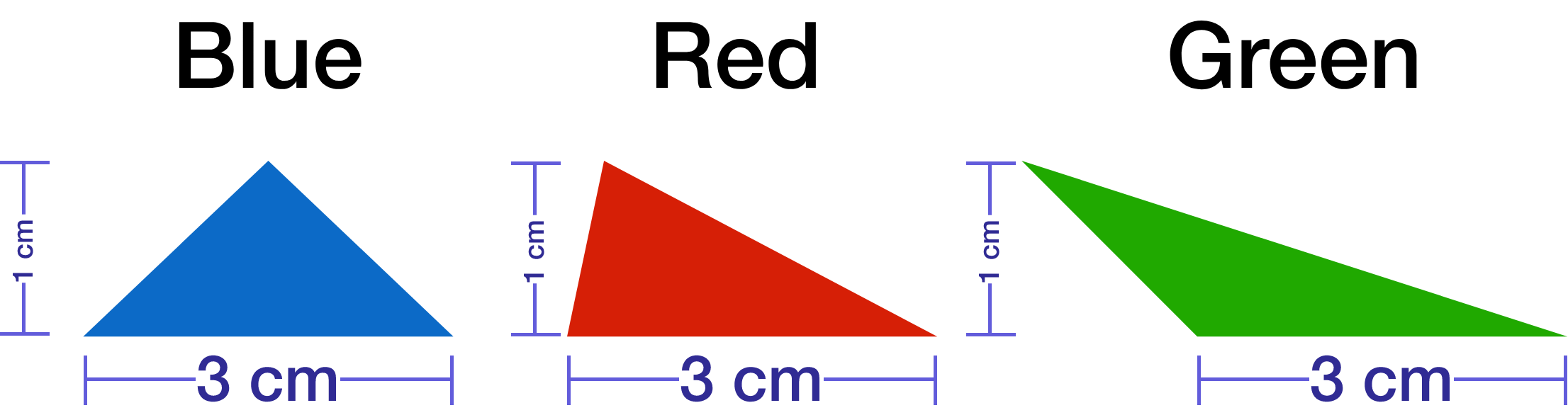Geometry

# Area Warmup

Area is a $$\text{ ________ }$$ dimensional measurement.

Is this statement true or false?

If the length and width of a rectangle double, then the area will double.

A rectangle and triangle have the same area and base length of 10. If the rectangle's height is 5, what is the height of the triangle?All triangles have the same height. Which has the greatest area?

A square and a triangle both have the same perimeter. Which has the greater area?

×